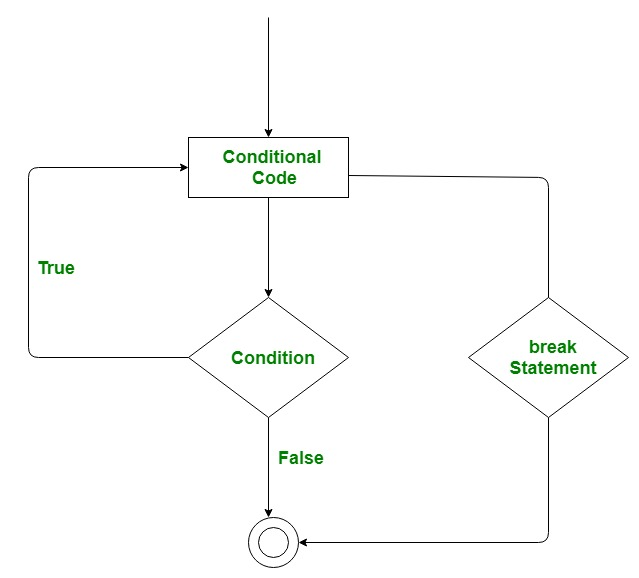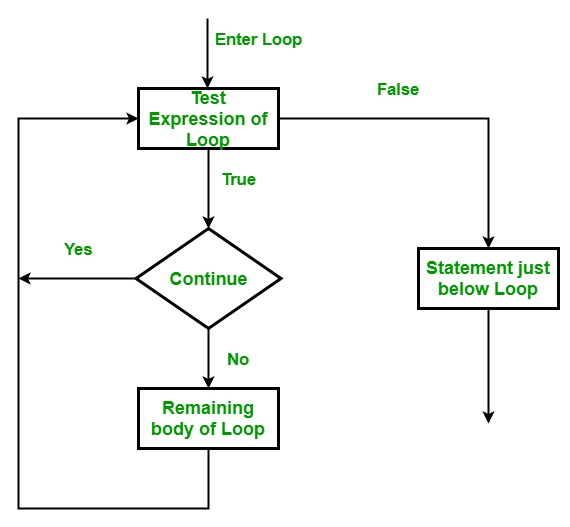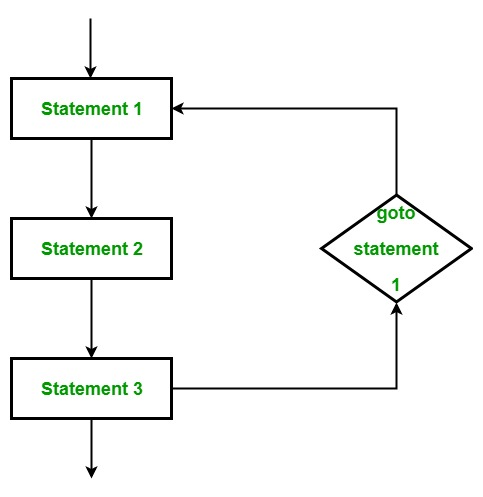Related Articles

# C# | Jump Statements (Break, Continue, Goto, Return and Throw)

• Difficulty Level : Medium
• Last Updated : 28 Oct, 2019

In C#, Jump statements are used to transfer control from one point to another point in the program due to some specified code while executing the program. There are five keywords in the Jump Statements:

• break
• continue
• goto
• return
• throw

#### break statement

The break statement is used to terminate the loop or statement in which it present. After that, the control will pass to the statements that present after the break statement, if available. If the break statement present in the nested loop, then it terminates only those loops which contains break statement.

Flowchart:Example:

 `// C# program to illustrate the``// use of break statement``using` `System;`` ` `class` `Geeks {`` ` `    ``// Main Method``    ``static` `public` `void` `Main()``    ``{`` ` `        ``// GeeksforGeeks is printed only 2 times``        ``// because of break statement``        ``for` `(``int` `i = 1; i < 4; i++) ``        ``{``            ``if` `(i == 3)``                ``break``;`` ` `            ``Console.WriteLine(``"GeeksforGeeks"``);``        ``}``    ``}``}`
Output:
```GeeksforGeeks
GeeksforGeeks
```

#### continue statement

This statement is used to skip over the execution part of the loop on a certain condition. After that, it transfers the control to the beginning of the loop. Basically, it skips its following statements and continues with the next iteration of the loop.Example:

 `// C# program to illustrate the``// use of continue statement``using` `System;`` ` `class` `Geeks {`` ` `    ``// Main Method``    ``public` `static` `void` `Main()``    ``{``         ` `        ``// This will skip 4 to print``        ``for` `(``int` `i = 1; i <= 10; i++) {`` ` `            ``// if the value of i becomes 4 then ``            ``// it will skip 4 and send the ``            ``// transfer to the for loop and ``            ``// continue with 5 ``            ``if` `(i == 4)``                ``continue``;`` ` `            ``Console.WriteLine(i);``        ``}``    ``}``}`
Output:
```1
2
3
5
6
7
8
9
10
```

#### goto statement

This statement is used to transfer control to the labeled statement in the program. The label is the valid identifier and placed just before the statement from where the control is transferred.Example:

 `// C# program to illustrate the``// use of goto statement``using` `System;`` ` `class` `Geeks {`` ` `    ``// Main Method``    ``static` `public` `void` `Main()``    ``{``        ``int` `number = 20;``        ``switch` `(number) {`` ` `        ``case` `5:``            ``Console.WriteLine(``"case 5"``);``            ``break``;``        ``case` `10:``            ``Console.WriteLine(``"case 10"``);``            ``break``;``        ``case` `20:``            ``Console.WriteLine(``"case 20"``);`` ` `            ``// goto statement transfer ``            ``// the control to case 5``            ``goto` `case` `5;`` ` `        ``default``:``            ``Console.WriteLine(``"No match found"``);``            ``break``;``        ``}``    ``}``}`
Output:
```case 20
case 5
```

#### return statement

This statement terminates the execution of the method and returns the control to the calling method. It returns an optional value. If the type of method is void, then the return statement can be excluded.

Example:

 `// C# program to illustrate the``// use of return statement``using` `System;`` ` `class` `Geeks {`` ` `    ``// creating simple addition function``    ``static` `int` `Addition(``int` `a)``    ``{`` ` `        ``// add two value and``        ``// return the result of addition``        ``int` `add = a + a;``        ` `        ``// using return statement``        ``return` `add;``    ``}`` ` `    ``// Main Method``    ``static` `public` `void` `Main()``    ``{``        ``int` `number = 2;`` ` `        ``// calling addition function``        ``int` `result = Addition(number);``        ``Console.WriteLine(``"The addition is {0}"``, result);``    ``}``}`
Output:
```The addition is 4
```

#### throw statement

This is used to create an object of any valid exception class with the help of new keyword manually. The valid exception must be derived from the Exception class.

Example:

 `// C# Program to illustrate the use ``// of throw keyword``using` `System;`` ` `class` `Geeks {``     ` `     ``// takinmg null in the string``     ``static` `string` `sub = ``null``;``         ` `    ``// method to display subject name    ``    ``static` `void` `displaysubject(``string` `sub1)``    ``{``        ``if` `(sub1  == ``null``)``            ``throw` `new` `NullReferenceException(``"Exception Message"``);``             ` `    ``}`` ` `// Main Method    ``static` `void` `Main(``string``[] args)``{``     ` `    ``// using try catch block to ``    ``// handle the Exception``    ``try``    ``{``         ` `        ``// calling the static method``        ``displaysubject(sub);``    ``}``     ` `    ``catch``(Exception exp)``    ``{``        ``Console.WriteLine(exp.Message );``    ``}                     ``}`` ` `}`
Output:
```Exception Message
```

My Personal Notes arrow_drop_up